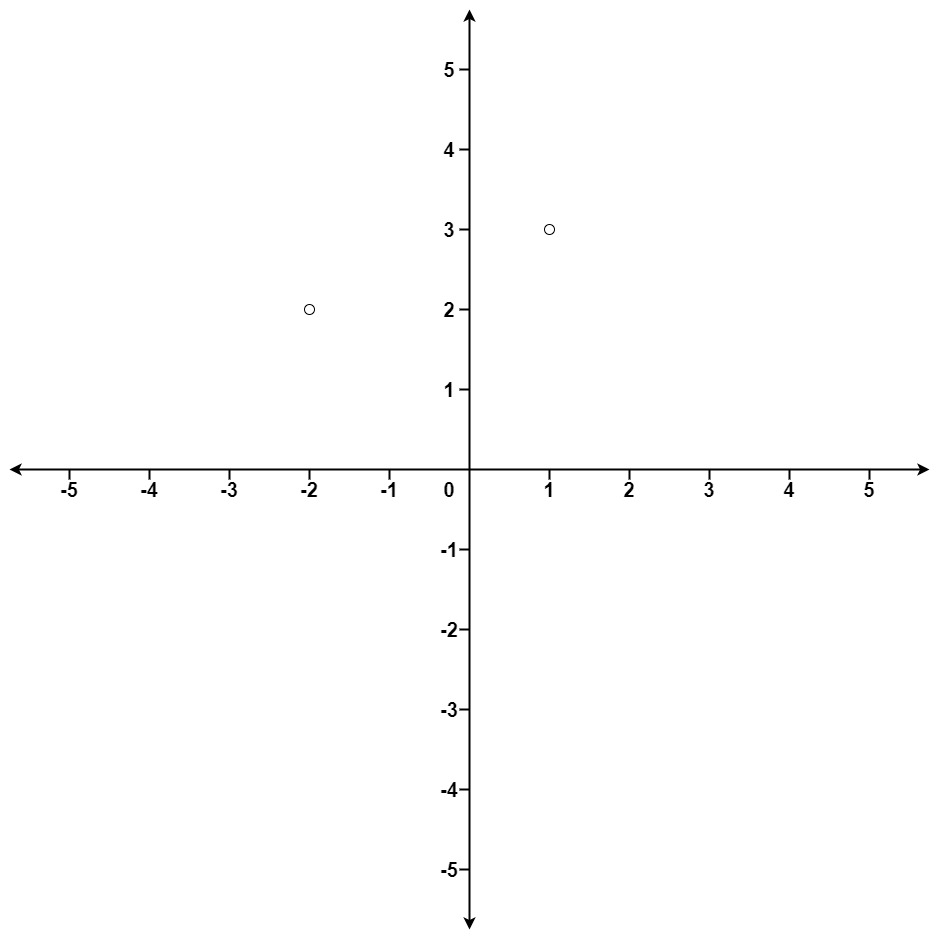# GeetCode Hub

Given an array of `points` where `points[i] = [xi, yi]` represents a point on the X-Y plane and an integer `k`, return the `k` closest points to the origin `(0, 0)`.

The distance between two points on the X-Y plane is the Euclidean distance (i.e., `√(x1 - x2)2 + (y1 - y2)2`).

You may return the answer in any order. The answer is guaranteed to be unique (except for the order that it is in).

Example 1:```Input: points = [[1,3],[-2,2]], k = 1
Output: [[-2,2]]
Explanation:
The distance between (1, 3) and the origin is sqrt(10).
The distance between (-2, 2) and the origin is sqrt(8).
Since sqrt(8) < sqrt(10), (-2, 2) is closer to the origin.
We only want the closest k = 1 points from the origin, so the answer is just [[-2,2]].
```

Example 2:

```Input: points = [[3,3],[5,-1],[-2,4]], k = 2
Output: [[3,3],[-2,4]]
Explanation: The answer [[-2,4],[3,3]] would also be accepted.
```

Constraints:

• `1 <= k <= points.length <= 104`
• `-104 < xi, yi < 104`

class Solution { public int[][] kClosest(int[][] points, int k) { } }